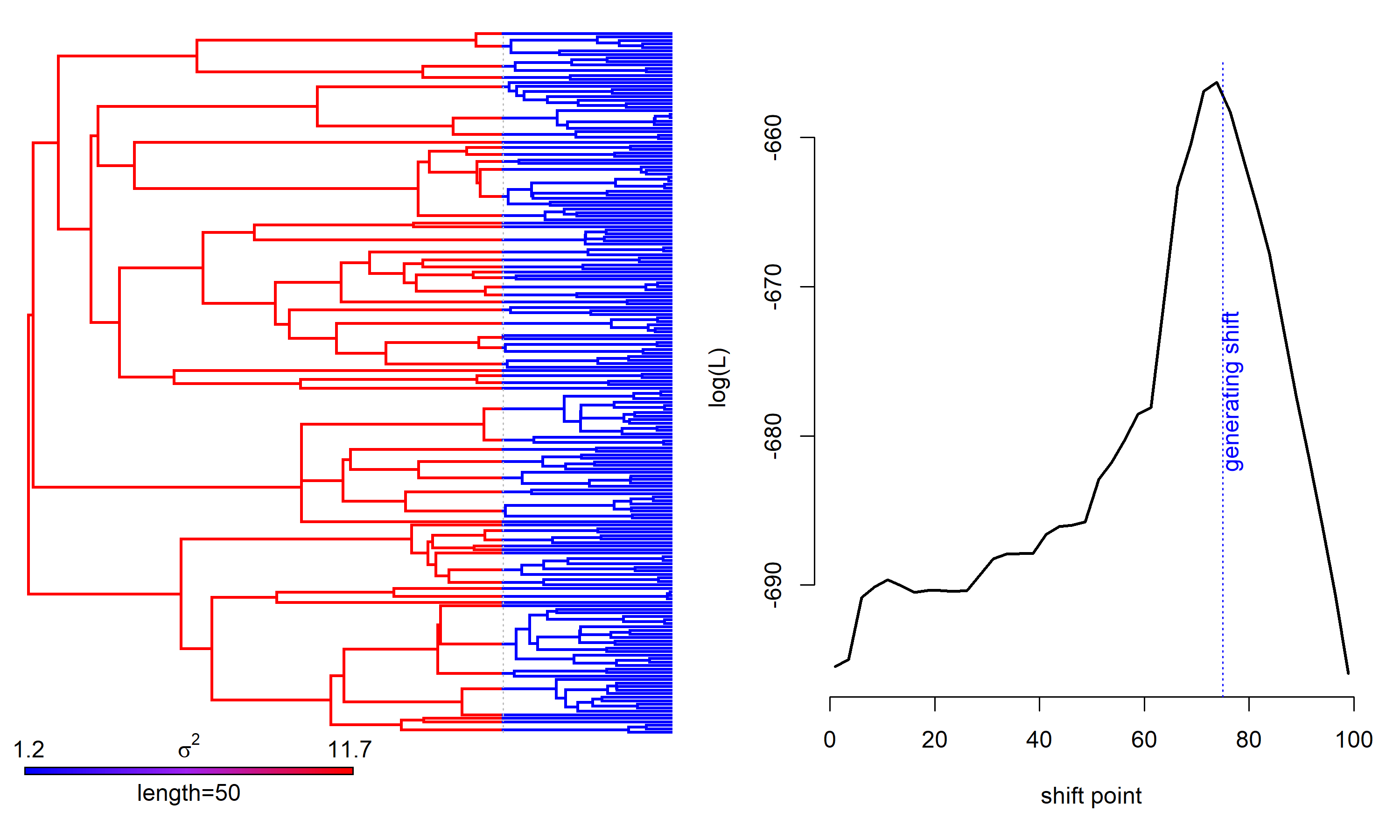## Tuesday, May 16, 2023

### Some updates to the function `rateshift` for detecting temporal shifts in the rate of phenotypic evolution on a phylogeny

A phytools user recently reported persistent optimization errors using the `phytools::rateshift` function for a large phylogenetic tree.

`rateshift` fits a model with one or multiple temporal rate shifts on the phylogeny, but whose positions are not known or hypothesized a priori by the investigator. For more information about this method, check out Chapter 5 of my recent Princeton University Press book with Luke Harmon (which, by chance, is 50% off from May 15-26).

I’ve made two small updates (1, 2) to `rateshift` with the hope of improving optimization performance as follows. (1) I wrapped our call to the optimizer in a `try` function call which (in theory) should cause `rateshift` to repeat any optimization iteration that fails, rather than returning just quitting. This is valuable because I’ve discovered that it’s not uncommon for `rateshift` to get into trouble on the likelihood surface and find parameter space where the likelihood is not defined or can’t be computed. (2) I use `foreach` (from the foreach CRAN package) to parallelize multiple optimization iterations across computer cores.

Just to see how this all works, let’s try simulating a tree & dataset with a single, known rate shift – and then fitting our model to these data to try to identify the temporal position of that shift.

To take advantage of these new options in `rateshift`, we should update the phytools package from its GitHub page using devtools.

``````## load phytools
library(phytools)
packageVersion("phytools")
``````
``````##  '1.9.2'
``````

Starting with simulation, I’ll use `make.era.map` to paint my generating regimes on the tree with a shift and rate 75% of the total tree height above the root.

``````## simulate phylogenetic tree
tree<-pbtree(n=200,scale=100)
## simulate rate-shift 75% of the total
## tree height above the root
tt<-make.era.map(tree,c(0,75))
sig2<-setNames(c(10,1),1:2)
x<-sim.rates(tt,sig2)
``````

Now I’ll run the original optimization iterations (`rateshift` does 10 by default) in serial, but with `try` added to help ensure our optimization doesn’t fail. I’ll wrap my function call in `system.time` so that I create a record of how long this takes.

``````system.time(
fit_serial<-rateshift(tree,x,nrates=2)
)
``````
``````## Optimization progress:
## |..........|
## Done.
``````
``````##    user  system elapsed
##   97.88    1.23  531.17
``````
``````fit_serial
``````
``````## ML 2-rate model:
##       s^2(1) se(1) s^2(2)  se(2) k   logL
## value  11.67 3.324  1.151 0.1375 4 -656.3
##
## Shift point(s) between regimes (height above root):
##         1|2 se(1|2)
## value 73.81   3.381
##
## Model fit using ML.
##
## Frequency of best fit: 0.7
##
## R thinks it has found the ML solution.
``````
``````system.time(
fit_parallel<-rateshift(tree,x,nrates=2,parallel=TRUE)
)
``````
``````## Opened cluster with 10 cores.
## Running optimization iterations in parallel.
## Done.
``````
``````##    user  system elapsed
##    1.00    0.28  154.72
``````
``````fit_parallel
``````
``````## ML 2-rate model:
##       s^2(1) se(1) s^2(2)  se(2) k   logL
## value  11.67 3.324  1.151 0.1375 4 -656.3
##
## Shift point(s) between regimes (height above root):
##         1|2 se(1|2)
## value 73.81   3.383
##
## Model fit using ML.
##
## Frequency of best fit: 0.7
##
## R thinks it has found the ML solution.
``````

Hopefully we see that our shift point is close to the generating value (75) and the same across our two different optimization routines.

Lastly, let’s plot our fitted model and a likelihood surface of the rate shift, just for fun.

``````par(mfrow=c(1,2))
plot(fit_parallel,ftype="off")
par(bty="n",mar=c(5.1,4.1,2.1,1.1))
lik2<-likSurface.rateshift(tree,x,nrates=2,
density=40)
``````
``````## Computing likelihood surface for 2-rate (1 rate-shift) model....
``````
``````## Done.
``````
``````par(fg="blue")
abline(v=75,lty="dotted")
text(x=75,y=mean(par()\$usr[3:4]),labels="generating shift",
srt=90,pos=1)
````````````par(fg="black")
``````

OK. I bet that worked even better than you expected.